# Kernel density plot in R

## Kernel density estimation

In order to create a kernel density plot you will need to estimate the kernel density. For that purpose you can use the `density` function and then pass the density object to the `plot` function.

``````# Data
set.seed(14012021)
data <- rnorm(200, mean = 4)

# Kernel density estimation
d <- density(data)

# Kernel density plot
plot(d, lwd = 2, main = "Default kernel density plot")``````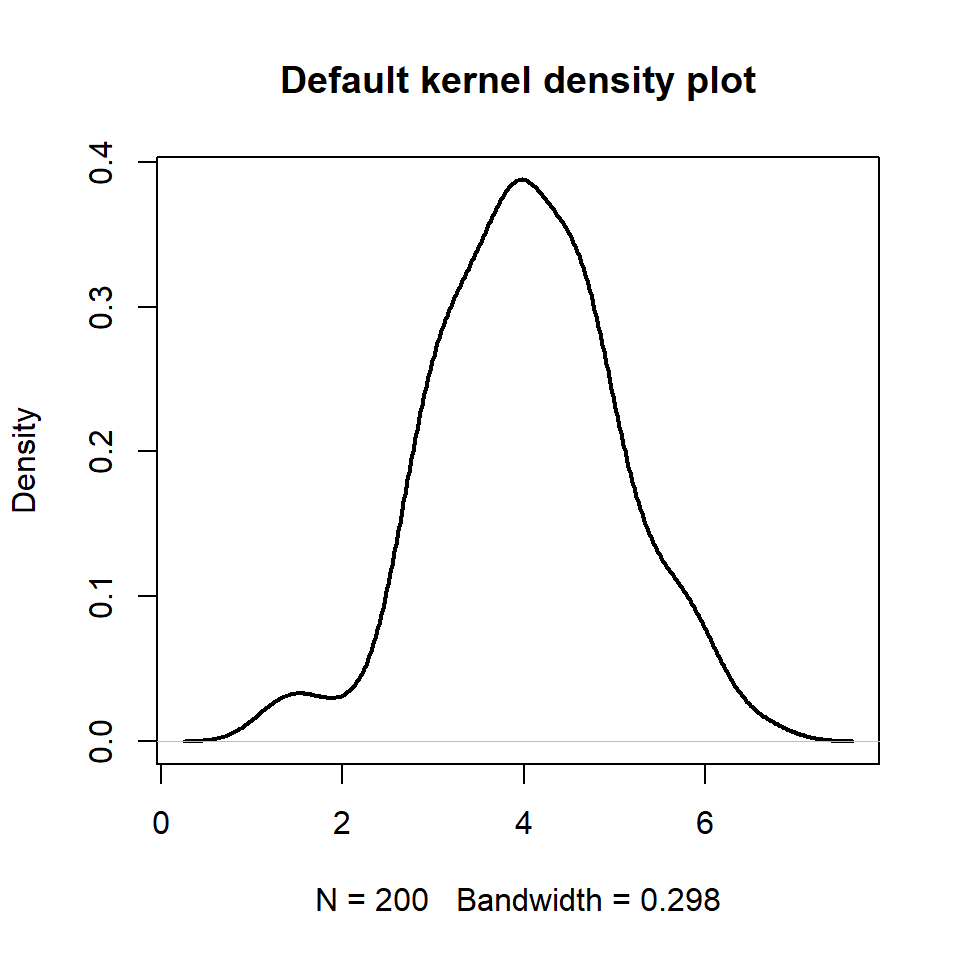## Kernel selection

The `kernel` argument of the `density` function uses the gaussian kernel by default (`kernel = "gaussian"`), but there are more kernel types available, such as `"rectangular"`, `"triangular"`, `"epanechnikov"`, `"biweight"`, `"cosine"` and `"optcosine"`. The selection will depend on your data, but in most scenarios the default value is the most recommended.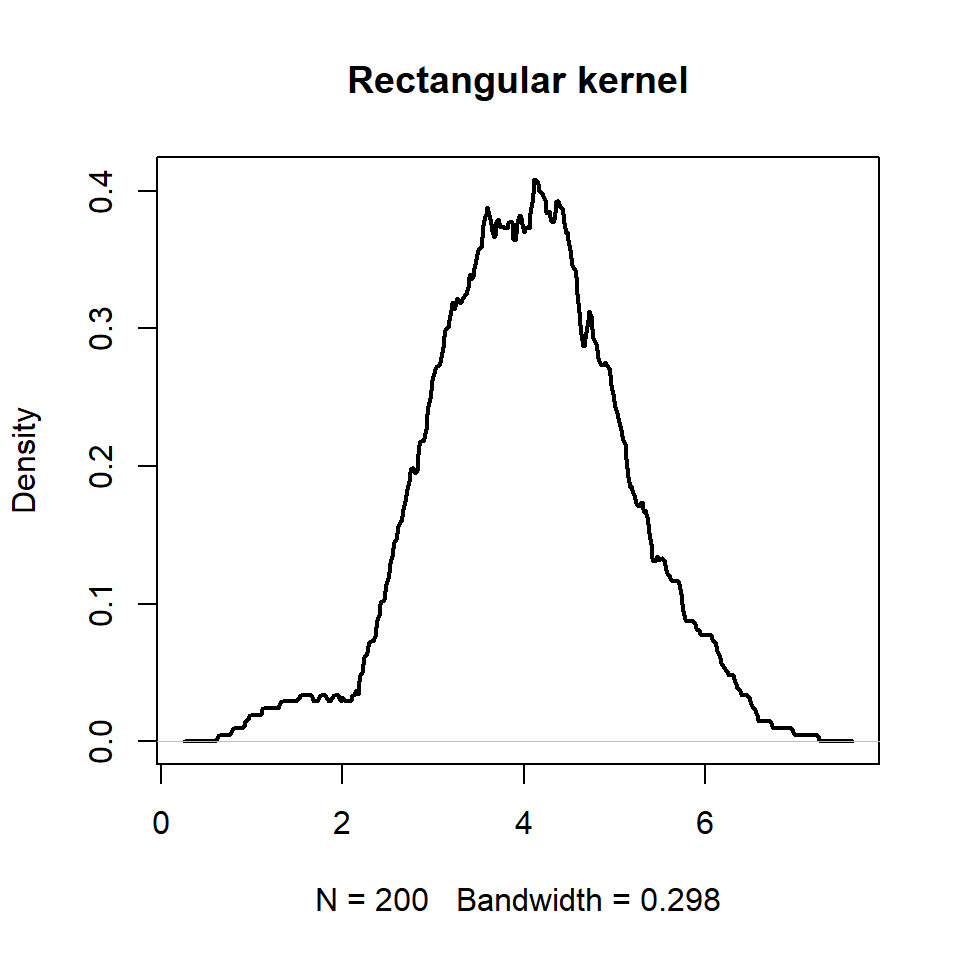Rectangular kernel

``````# Data
set.seed(14012021)
data <- rnorm(200, mean = 4)

# Kernel density estimation
d <- density(data,
kernel = "rectangular")

# Kernel density plot
plot(d, lwd = 2, main = "Rectangular kernel")``````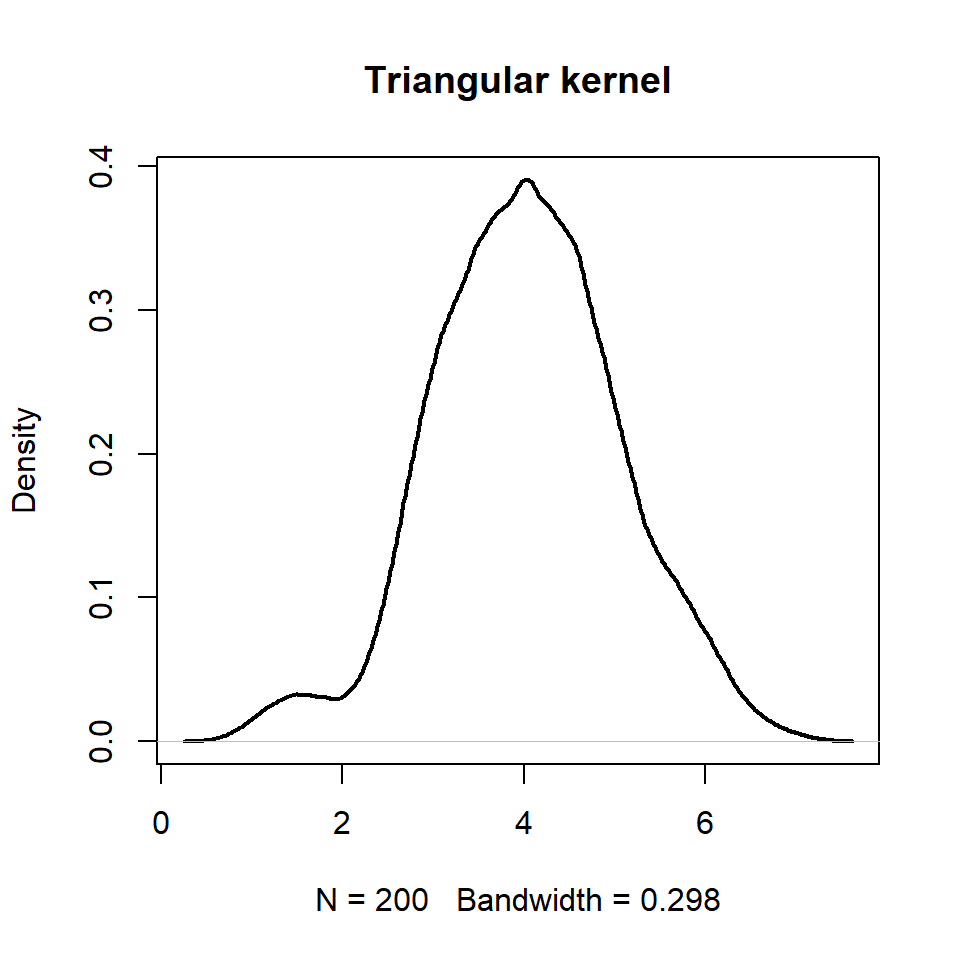Triangular kernel

``````# Data
set.seed(14012021)
data <- rnorm(200, mean = 4)

# Kernel density estimation
d <- density(data,
kernel = "triangular")

# Kernel density plot
plot(d, lwd = 2, main = "Triangular kernel")``````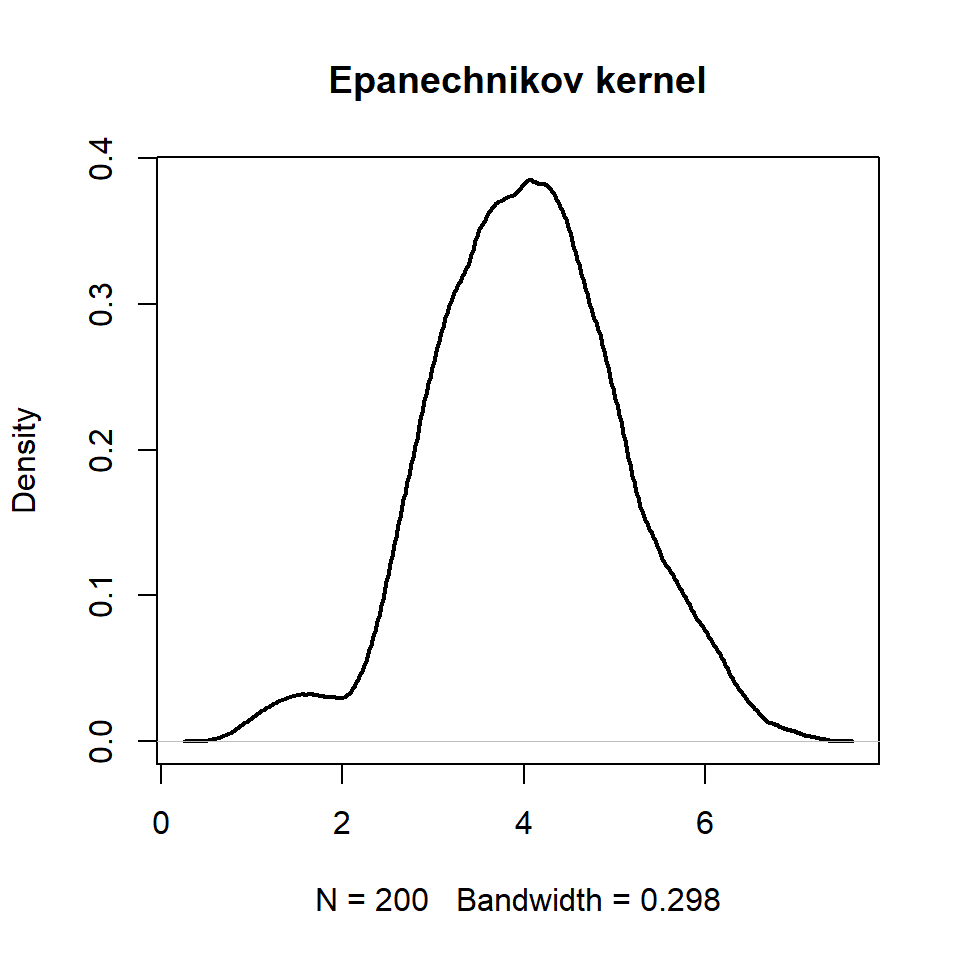Epanechnikov kernel

``````# Data
set.seed(14012021)
data <- rnorm(200, mean = 4)

# Kernel density estimation
d <- density(data,
kernel = "epanechnikov")

# Kernel density plot
plot(d, lwd = 2, main = "Epanechnikov kernel")``````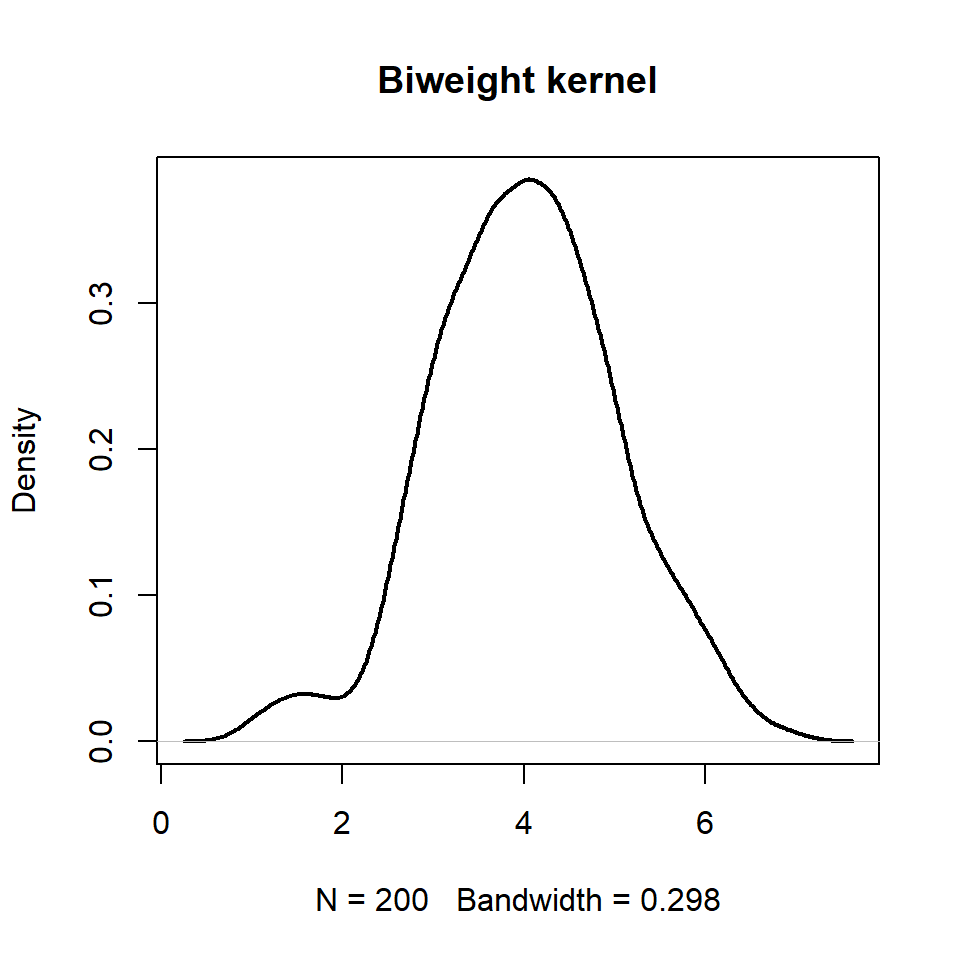Biweight kernel

``````# Data
set.seed(14012021)
data <- rnorm(200, mean = 4)

# Kernel density estimation
d <- density(data,
kernel = "biweight")

# Kernel density plot
plot(d, lwd = 2, main = "Biweight kernel")``````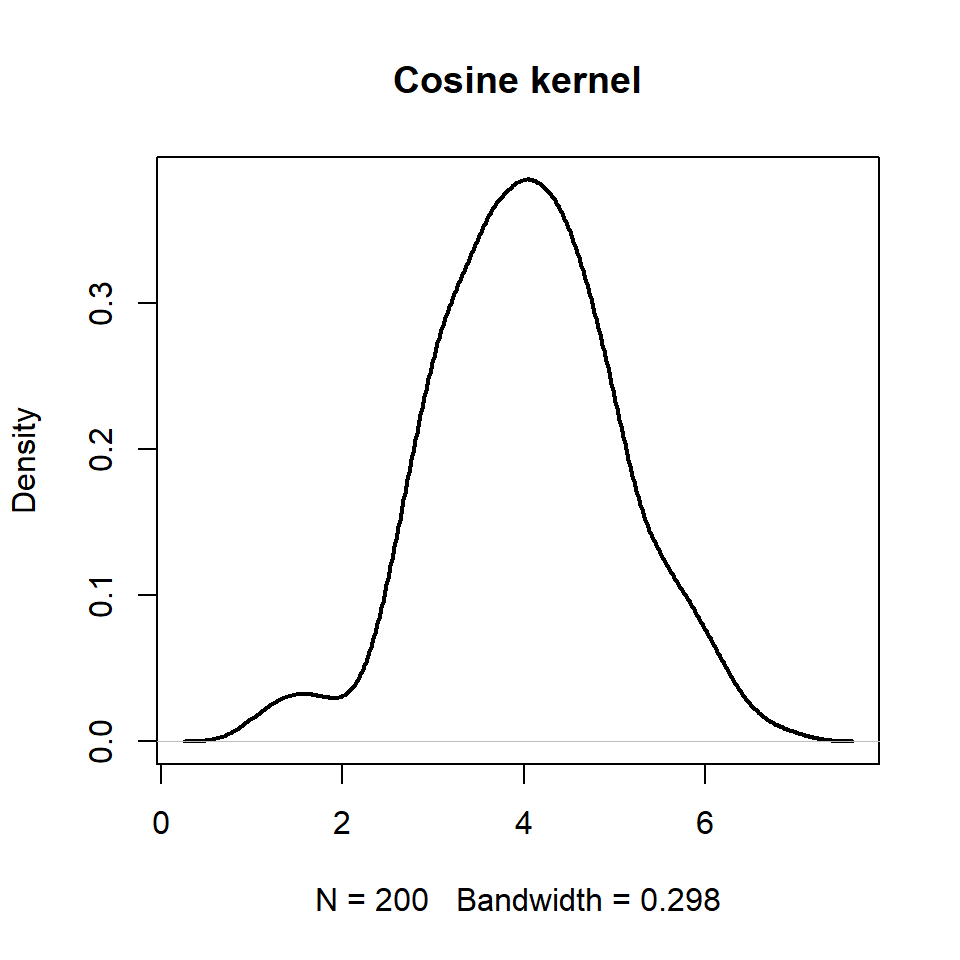Cosine kernel

``````# Data
set.seed(14012021)
data <- rnorm(200, mean = 4)

# Kernel density estimation
d <- density(data,
kernel = "cosine")

# Kernel density plot
plot(d, lwd = 2, main = "Cosine kernel")``````

## Bandwidth selection

The `bw` argument of the `density` function allows changing the smoothing bandwidth used. You can pass either a value or a string giving a selection rule or a function. The default value is `"nrd0"` (or `bw.nrd0(.)`), which implements a rule-of-thumb approach. Other available options are:

Rule-of-thumb variation given by Scott (1992)

`"nrd"` or `bw.nrd(.)`

``````# Data
set.seed(14012021)
data <- rnorm(200, mean = 4)

# Kernel density estimation
d <- density(data,
bw = "nrd")

# Kernel density plot
plot(d, lwd = 2, main = "nrd bandwidth")``````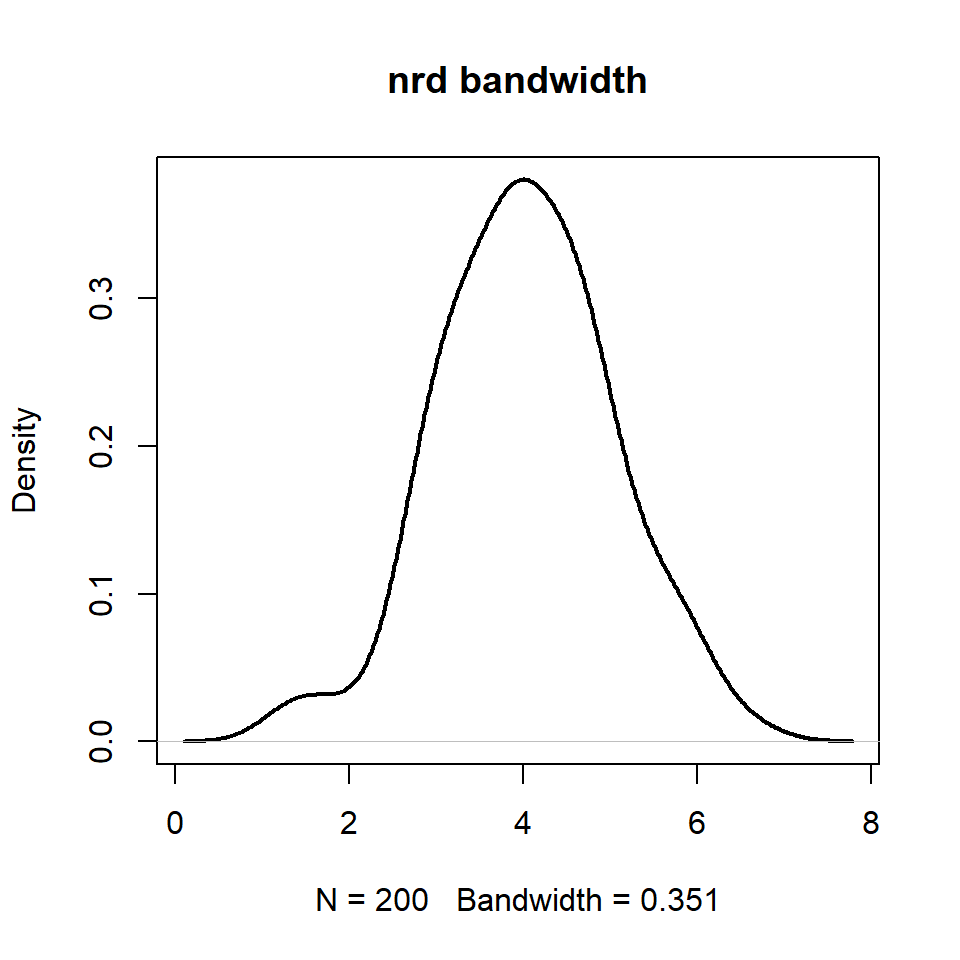Unbiased cross-validation

`"ucv"` or `bw.ucv(.)`

``````# Data
set.seed(14012021)
data <- rnorm(200, mean = 4)

# Kernel density estimation
d <- density(data,
bw = "ucv")

# Kernel density plot
plot(d, lwd = 2, main = "ucv bandwidth")``````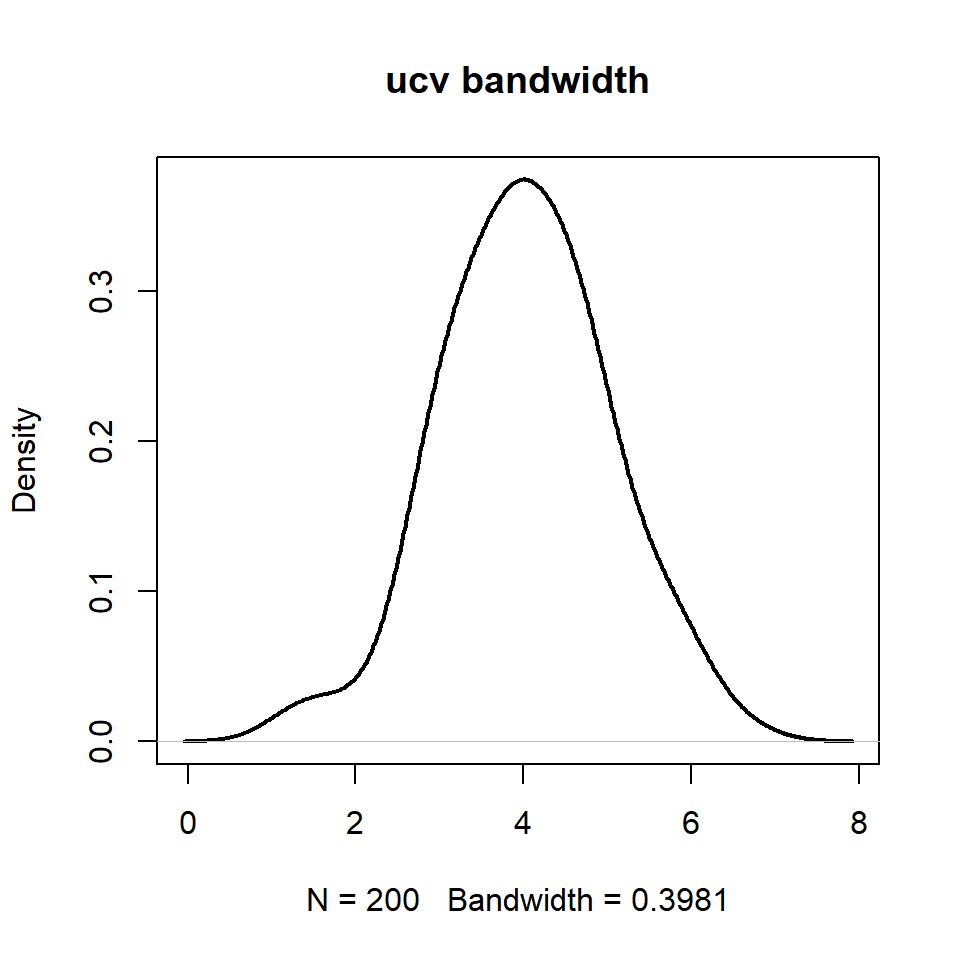Biased cross-validation

`"bcv"` or `bw.bcv(.)`

``````# Data
set.seed(14012021)
data <- rnorm(200, mean = 4)

# Kernel density estimation
d <- density(data,
bw = "bcv")

# Kernel density plot
plot(d, lwd = 2, main = "bcv bandwidth")``````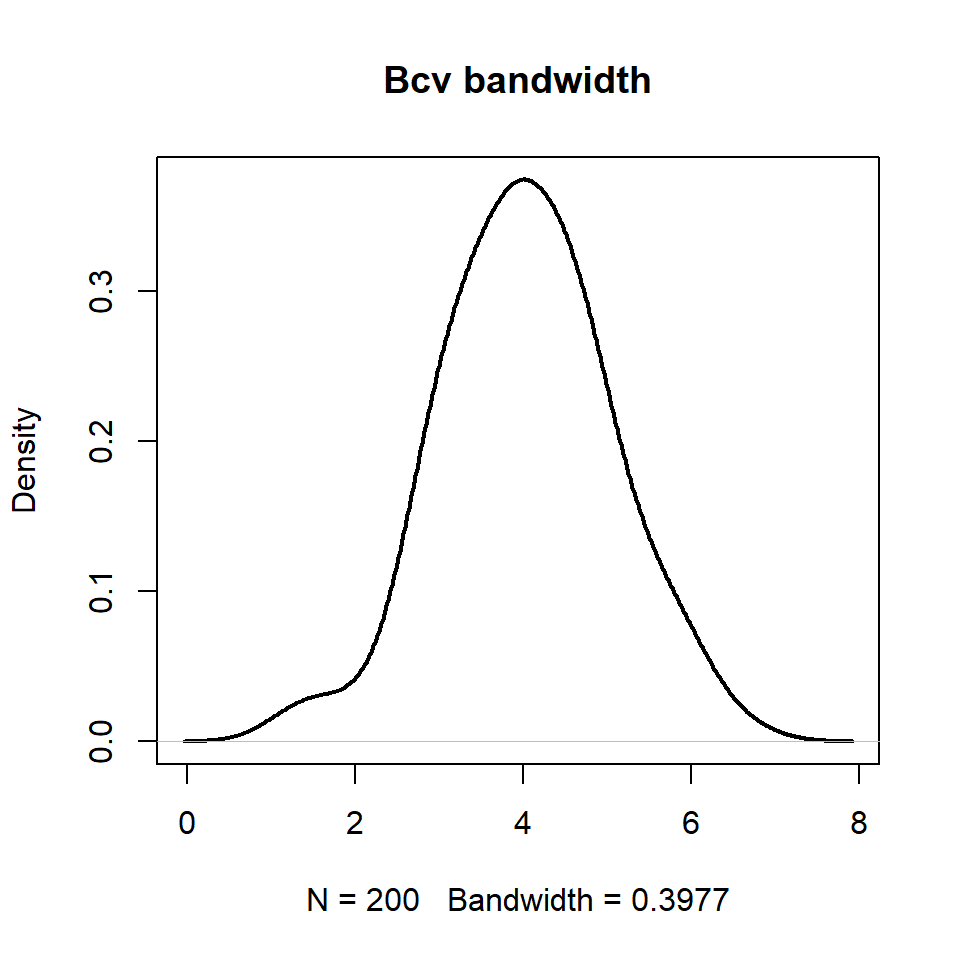Methods by Sheather & Jones (1991)

`"SJ"` or `bw.SJ(.)`

``````# Data
set.seed(14012021)
data <- rnorm(200, mean = 4)

# Kernel density estimation
d <- density(data,
bw = "SJ")

# Kernel density plot
plot(d, lwd = 2, main = "SJ bandwidth")``````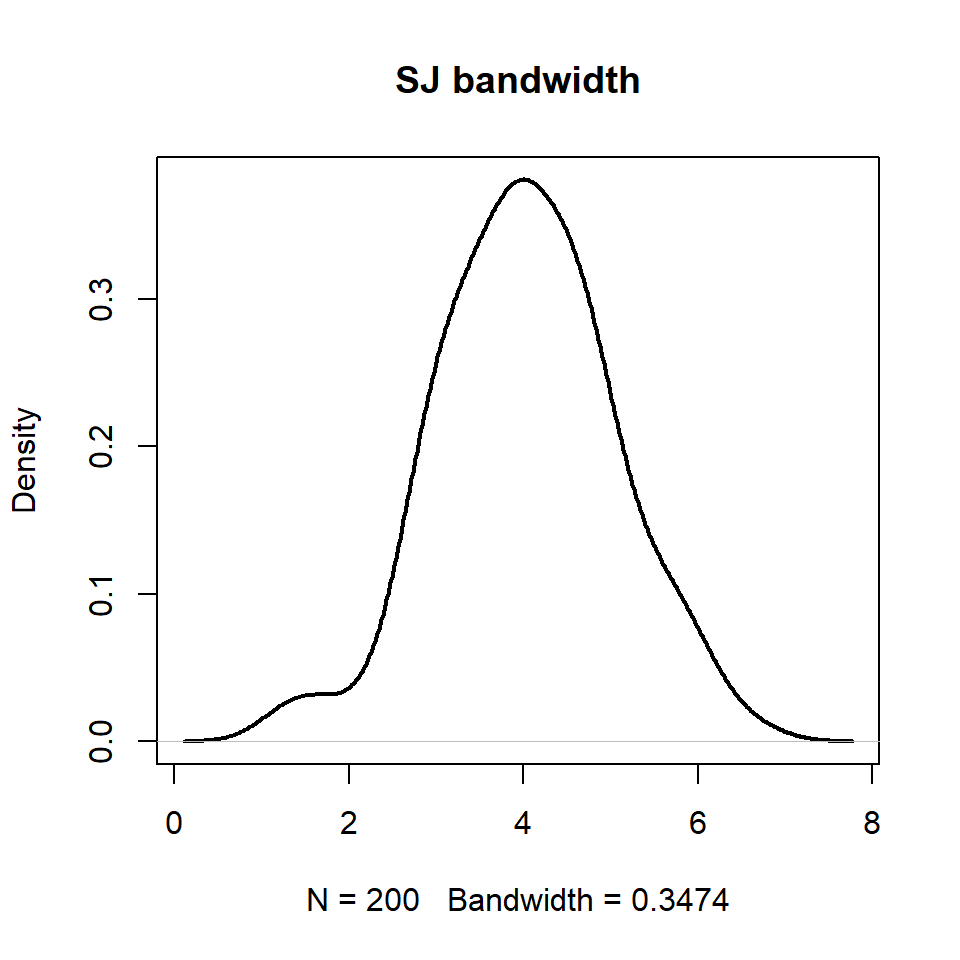The bandwidth must be chosen carefully. A small bandwidth will create a very overfitted curve while a too big bandwidth will create an oversmoothed curve.